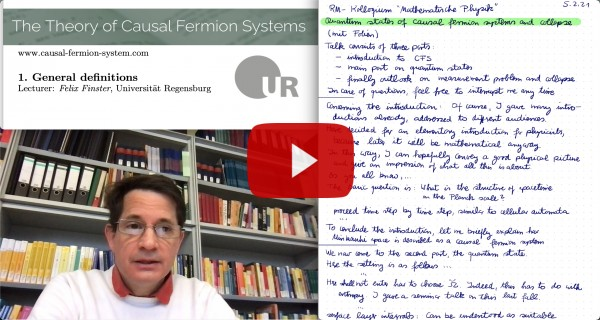# The Theory of Causal Fermion Systems

## The Dynamical Wave EquationA causal fermion system gives rise to a distinguished family of wave functions in spacetime, the physical wave functions. When perturbing the system while preserving the Euler-Lagrange equations, also the physical wave equations are perturbed. In [FKO21] it is shown that, under general assumptions, a class of wave functions obtained in this way satisfy the dynamical wave equation

$\int_M Q^\text{dyn}(x,y)\: \psi(y)\: d\rho(y) = 0 \:.$

These wave functions form a Hilbert space $\H_\rho$ which extends the Hilbert space $\H$ of the causal fermion system. It generalizes the solution space of the Dirac equation to the setting of causal fermion systems. The scalar product on $\H_\rho$ can be represented at any time by a surface layer integral denoted by $\langle .|. \rangle^t_\rho$.

The conservation law corresponding to the symmetry described by unitary transformations generalizes to a conserved surface layer integral for solutions of the dynamical wave equation. It takes the form (for details see [FKO21])

\begin{align*}
\la \psi | \phi \ra^\Omega_\rho = -2i \,\bigg( \int_{\Omega} d\rho(x) \int_{M \setminus \Omega} d\rho(y) &- \int_{M \setminus \Omega} d\rho(x) \int_{\Omega} d\rho(y) \bigg) \\
&\times\: \Sl \psi(x) \:|\: Q^\text{dyn}(x,y)\, \phi(y) \Sr_x \:.
\end{align*}